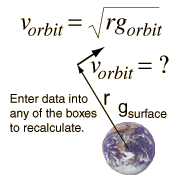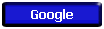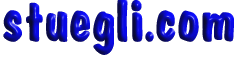# Orbital Velocity caclulatorAbove the earth's surface at a height of h =m    = x 106 m, which is the same as a radius r = x earth radius, agravity at radius r = m/s2 = x gearth surface  (9.8 m/s2) At the specified orbit radius, the velocity required for orbit is vtangential =m/s=km/h=mi/h. The period of the orbit is minutes = hours.Copyright © 2005 -  S. B. EglICopyright © 2005 -  S. B. EglI